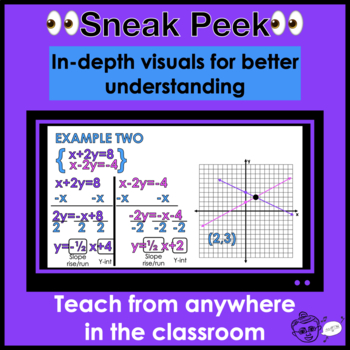# Algebra 1 PowerPoint Lessons

Rated 4.76 out of 5, based on 42 reviews
42 Ratings8th - 10th
Subjects
Formats Included
• Zip
\$172.80
List Price:
\$192.00
You Save:
\$19.20
Bundle
\$172.80
List Price:
\$192.00
You Save:
\$19.20
Bundle
Report this resource to TPT

#### Products in this Bundle (64)

showing 1-5 of 64 products

### Description

Do you need Algebra 1 PowerPoint lessons with notes that are ready-to-go? This bundle includes lessons with examples that are animated step-by-step, allowing you to teach from anywhere in the classroom!

Each lesson in this bundle includes notes, a video lesson on YouTube and is fully editable.

This product includes: equations, inequalities, absolute value, functions, linear functions, graphing, writing equations, systems, linear inequalities, exponentials, polynomials, factoring & quadratics.

My Product Includes

• 60+ lessons
• Printable notes for each presentation

Topics included:

Equations

• 1 One Step Equations
• 2 Two Step Equations
• 3 Multi Step Equations
• 4 Multi Step Equations w/ Variables on both sides
• 5 No solution Infinitely Many Solutions

Inequalities

• 6 One Step Inequalities
• 7 Two Step Inequalities
• 8 Multi Step Inequalities
• 9 Multi Step Inequalities w/ Variables on both sides
• 10 Compound (and/or) Inequalities

Absolute Value

• 11 Absolute Value Equations
• 12 Absolute Value Inequalities
• 13 Absolute Value Graphs (soon)

Functions

• 14 Is it a function?
• 15 Domain and Range
• 16 Evaluating Functions (graphs tables and equations)
• 17 Rearranging Formulas (literal equations) ::soon::

Intro to Linear Functions

• 18 What is a Linear Function
• 19 Making Tables for Linear Function
• 20 Graphing Using X & Y Intercepts
• 21 What is an Intercept?
• 22 Standard Form & Point Slope Form to Slope Intercept form (isolating y)

Slopes

• 23 Find the Slope given a graph (rise & run)
• 24 Find the Slope given an equation
• 25 Find the slope given 2 points (slope formula)

Graphing Linear Functions

• 26 Graphing in Point Slope Form
• 27 Graphing in Slope Intercept Form

Writing Linear Equations

• 28 With 2 Points
• 29 With a Point and a Slope
• 30 With a Y-Int and a Slope

Systems

• 31 Graphing
• 32 Substitution
• 33 Elimination
• 34 Word Problems
• 35 Classifying Systems

Linear Inequalities

• 36 Graphing Linear Inequalities
• 37 Graphing Systems of Linear Inequalities

Exponentials

• 38 Rules of Exponents
• 39 What is an Exponential Function
• 40 Characteristics of Exponential Graphs
• 41 Compound Interest
• 42 Exponential Growth & Decay
• 43 Graphing Basic Exponential Functions

Polynomials

• 44 What is a polynomial
• 45 Adding & Subtracting Polynomials
• 46 Multiplying Polynomials
• 47 Polynomial Special Products

Factoring

• 48 GCFs
• 49 a=1
• 50 a>1
• 51 Perfect Square Trinomials
• 52 The Difference of 2 Squares

• 54 Completing the Square
• 55 Solve by Square Roots
• 57 By Factoring
• 58 Vertical Motion Model (word problems)

• 59 What is a Quadratic
• 61 Graphing Quadratics in Standard Form
• 62 Graphing Quadratics in Vertex Form
• 64 Compare Quadratic, Exponential and Linear Functions

Check out these other products too!
Algebra 1 Powerpoint Keynote Presentation Bundle
Algebra 2 Powerpoint Keynote Bundle
Math Activities Bundle
Alg 2 Assessment Bundle- Microsoft Forms
Algebra 2 Notebook Guided Notes

Let’s hang!
Find me on:
Instagram
Website

Total Pages